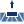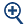# View Seam Weld Reactions

Display a table of seam weld resultant forces and stresses.

Total contact forces and average stresses are displayed for each weld but seam weld resultant forces and stresses at the throat can also be evaluated per unit length. The number of data points for this evaluation is determined automatically by the length of the weld. Resultant forces and stresses per unit length can be used with seam weld design standards, specifically Eurocode 3 (EN 1993-1-8: Eurocode 3: Design of Steel Structures, Section 4.5.3).

## Force/Moment

1. On the Project Tree, open the Analysis Workbench.
2. On the workbench toolbar, select(Weld forces) > Seam weld reactions.
3. In the Seam weld reaction dialog, select the Force/Moment tab.
4. Click Evaluate to calculate Force/Moment components along the weld length for all welds. This estimates the peaks (maximum and minimum) among all the welds in the model.
5. Set the desired threshold value as a failure criterion for Force per unit length.
6. Click Validate to see the status of each weld after evaluation.
7. Select any column header to sort the table.
8. Select any row to display a Force and Moment summary along with their vectors in graphics.
9. Optional: Use(Zoom) to focus the modeling window on the chosen weld.
10. View a plot of a seam weld's reaction per unit length over the length of the weld.
1. For Reaction type, select the reaction type of interest from the drop-down menu.
2. Select Show.
The selected reaction is plotted in a new window.
11. In the plot window, select Save as to save results as an image or text file.

## Stresses

1. On the Project Tree, open the Analysis Workbench.
2. On the workbench toolbar, select(Weld forces) > Seam weld reactions.
3. In the Seam weld reaction dialog, select the Stresses tab.
4. Click Evaluate to calculate various stress components along the weld length for all welds.
5. Set the desired threshold value as a failure criterion for the stresses (normal stress, shear stress, and equivalent stress).
Equivalent stress is calculated based on the below equation.(1) ${\left[{\sigma }_{\perp }{}^{2}+3\left({\tau }_{\perp }{}^{2}+{\tau }_{\parallel }{}^{2}\right)\right]}^{0.5}$ Equivalent stress should be lesser than the standard values calculated. An example based on EN 1993-1-8 Eurocode 3 gives the following equation.(2) ${\left[{\sigma }_{\perp }{}^{2}+3\left({\tau }_{\perp }{}^{2}+{\tau }_{\parallel }{}^{2}\right)\right]}^{0.5}\le {f}_{u}/\left({\beta }_{w}{\gamma }_{M2}\right)$ Where,
${\sigma }_{\perp }$
Normal stress perpendicular to the throat
${\tau }_{\perp }$
Shear stress (in the plane of the throat) perpendicular to the axis of the weld
${\tau }_{\parallel }$
Shear stress (in the plane of the throat) parallel to the axis of the weld
For the standard values calculated based on the Eurocode example, the terminologies are mentioned below where,
${f}_{u}$
Nominal ultimate tensile strength of the weaker part joined
${\beta }_{w}$
Appropriate correlation factor
6. Click Validate to see the status of each weld after evaluation.
7. For average stress calculations, the ability to divide each weld into further sections can be activated by checking Include weld section stresses. Then you can enter a value for Section length to throat thickness ratio.
This value ensures a weld is not constrained to a particular welding standard.
8. Select any column header to sort the table.
9. Optional: Use(Zoom) to focus the modeling window on the chosen weld.
10. View a plot of a seam weld's stresses per unit length over the length of the weld.
1. For Reaction type, select a stress of interest from the drop-down menu.
2. Select Show.
The selected stress along the length of weld is plotted in a new window.
11. In the plot window, select Save as to save results as an image or text file.